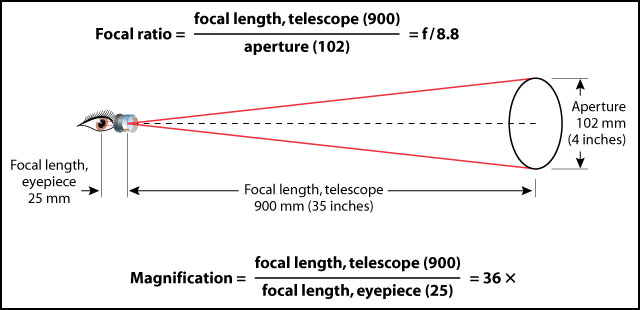How to calculate what you need to know about your telescopes, oculars, and binoculars.

I am about as far from being a mathematician as it is possible to get. However, sometimes it is good to know how to calculate some of the numbers commonly mentioned by telescope owners and in telescope articles. (Binoculars are just two small telescopes linked together, so the same formulas work for them, too.) Armed with a simple calculator and the following formulas, even I can work out the vital statistics of my telescopes and eyepieces.

Most of the values you need to know to work these formulas, such as prime focal length, focal ratio and clear aperture, are usually printed right on the optical tubes of astronomical telescopes, binoculars and the barrel of eyepieces. If they are not, the manufacturer's web site usually has the basic numbers, as should the instrument's Owners Manual. To illustrate the various formulas below, I will use a telescope with a 100mm (4") clear aperture and a prime focal length of 1000mm.

## Magnification

The magnification of an astronomical telescope changes with the eyepiece used. It is calculated by dividing the focal length of the telescope (usually marked on the optical tube) by the focal length of the eyepiece (both in millimeters). Thus:

TELESCOPE FOCAL LENGTH / OCULAR FOCAL LENGTH = MAGNIFICATION

For example, a telescope with a 1000mm focal length using a 10mm ocular is operating at 100x magnification (1000/10=100).

### Focal Ratio (f/stop)

The focal ratio, or f/stop, of any lens system (including telescopes), is computed by dividing the focal length by the clear aperture (usually expressed in millimeters). In other words, the focal ratio is the ratio of the focal length and clear aperture. Thus:

TELESCOPE FOCAL LENGTH / CLEAR APERTURE = FOCAL RATIO

For example, a telescope with a focal length of 1000mm and a 100mm (4") clear aperture has a focal ratio of f/10 (1000/100=10).An example calculation of focal ratio and magnification for a scope with a 25 mm focal length, eyepiece, 102 mm aperture, and 900 mm focal length, telescope. Always make sure you're using the same units!

## True Field of View

There are two ways to calculate the true field of view (FOV) in degrees of a telescope and eyepiece combination. The easy way and the method I use is to divide the apparent field of view (AFOV) of the ocular by the magnification of the system. The AFOV for almost all eyepieces is provided by the manufacturer and it is easy to derive the magnification of any telescope/ocular combination. Thus:

AFOV / MAGNIFICATION = FOV

For example, a 25mm Plossl eyepiece generally has an AFOV of 50-degrees. Used in a telescope with a 1000mm prime focal length, the magnification is 40x. The true field of view is therefore 1.25-degrees (50/40=1.25).

The other formula for calculating FOV in degrees involves dividing the eyepiece field stop diameter by the prime focal length of the telescope and multiplying the result by the constant of 57.3. Unfortunately, most ocular manufacturers do not provide a field stop diameter for their eyepieces; one company that does is Tele Vue. Thus:

OCULAR FIELD STOP DIAMETER / TELESCOPE FOCAL LENGTH x 57.3 = FOV

A Tele Vue 25mm Plossl has a field stop diameter of 21.2mm. Used in our 1000mm focal length telescope this formula produces a FOV of slightly over 1.2 degrees (21.2 / 1000 = 0.0212 × 57.3 = 1.21476). The results produced by the two formulas are very similar, but not quite identical. I do not know which method is more accurate, but both are close enough for practical purposes.

## Power per Inch

This is good to know, because it is a truism among amateur astronomers that the power per inch (PPI) figure of a telescope and ocular should not exceed 50 PPI in excellent seeing conditions. In average seeing conditions, I figure about 30 PPI as a practical maximum.

PPI can be calculated by dividing the magnification of the telescope and eyepiece combination by the telescope's clear aperture in inches (1 inch = approximately 25mm). Thus:

MAGNIFICATION / APERTURE (IN.) = PPI

For example, a 100mm clear aperture is approximately 4", so such a telescope operating at 100x magnification is at 25 PPI (100/4=25).

## Exit Pupil

The exit pupil is the diameter of the "light pencil" that emerges from the eyepiece. The pupil of fully dark-adapted human eye can dilate to about 7mm diameter, so an exit pupil in excess of 7mm is passing more light than the eye can accept. On the other hand, as the exit pupil decreases below 7mm, lack of light becomes the basic limiting factor to what you can see at night. Exit pupils of less than about 0.5mm are so small and pass so little light to the eye that they are functionally useless. Actually, I like exit pupils of at least 1.0mm for decent viewing.

Exit pupil can be calculated by dividing the telescope's clear aperture (in millimeters) by the magnification produced by the ocular in use. Thus:

APERTURE / MAGNIFICATION = EXIT PUPIL

For example, our 100mm clear aperture telescope with a 10mm ocular is operating at 100x magnification and therefore has a 1.0mm exit pupil (100/100=1)

Another way to calculate exit pupil is to divide the eyepiece focal length in millimeters by the telescope's focal ratio (f/stop).

OCULAR FOCAL LENGTH / TELESCOPE FOCAL RATIO = EXIT PUPIL

Thus, a 10mm ocular in our f/10 (100mm clear aperture and 1000mm focal length) telescope has a 1.0mm exit pupil (10/10=1). Either formula results in the same answer.

This article originally appeared on Chuck Hawks' website and is reposted with the author's permission. Images added from Sky & Telescope's library. Click here for a handy calculator and an explanation of terms.